## 6.2.8 Estimation of Overall Efficiency

To get reasonable count statistics approximately one particle per second should be registered. The overall efficiency is mainly influenced by the efficiency of the neutralizer and by the reflection properties of the conversion surface. Additional losses are due to the small size of the conversion surface samples and because the extraction lens does not focus in a direction perpendicular to the plane by the conversion surface normal and the impinging neutral beam. The number of counts per second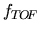to be expected is given by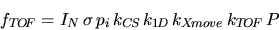(6.13)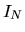denotes the neutral flux from the neutralizer,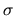the reflection efficiency of the CS,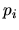the ionization efficiency of the CS,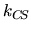the loss due to the non-rectangular shape of the CS including losses due to the clamps holding the CS in place,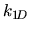the loss due to the fact that the lens focuses only in one direction.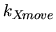denoted the loss due to the movable lens. The neutral beam remains fixed while the lens with the CS is movable to allow for energy selection at the lens exit aperture with the TOF entry slit. As the lens was moved approximately 25mm and the typical sample also had a diameter of 25mm the neutral beam had to be enlarged such that the CS was covered all the time by the neutral beam footprint. This implied that at least half of the neutral beam hit some lens structure instead of the CS.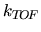would account for the geometrical coverage of the exit slit by the TOF entry slit and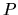denotes the detection efficiency of the CODIF at particle energies around 14keV.

Using Equation 6.13 with the values for the diamond sample (low energy neutral atomic oxygen,= 0.2,= 0.15,= 0.5,= 0.25,= 0.5,= 0.5, and= 0.7) and a neutral beam intensityof 6000 particles per second produced by a 110eV CO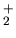primary beam (see Section 6.2.2), about 4 ions should be detected per second. The observed value forwas 0.3 to 1 ions per second. According to  CO-molecules are very likely to survive the collision with the CS without dissociation. The oxygen in the CO molecule would then be lost for this calculation. Thus the neutral oxygen atom beam intensityin the above example is only half the value (3000 oxygen atoms per second) yielding 2 oxygen ions per second to be detected with the TOF. Given the large uncertainties in the above calculations the estimated and the measured count rates are identical.

March 2001 - Martin Wieser, Physikalisches Institut, University of Berne, Switzerland